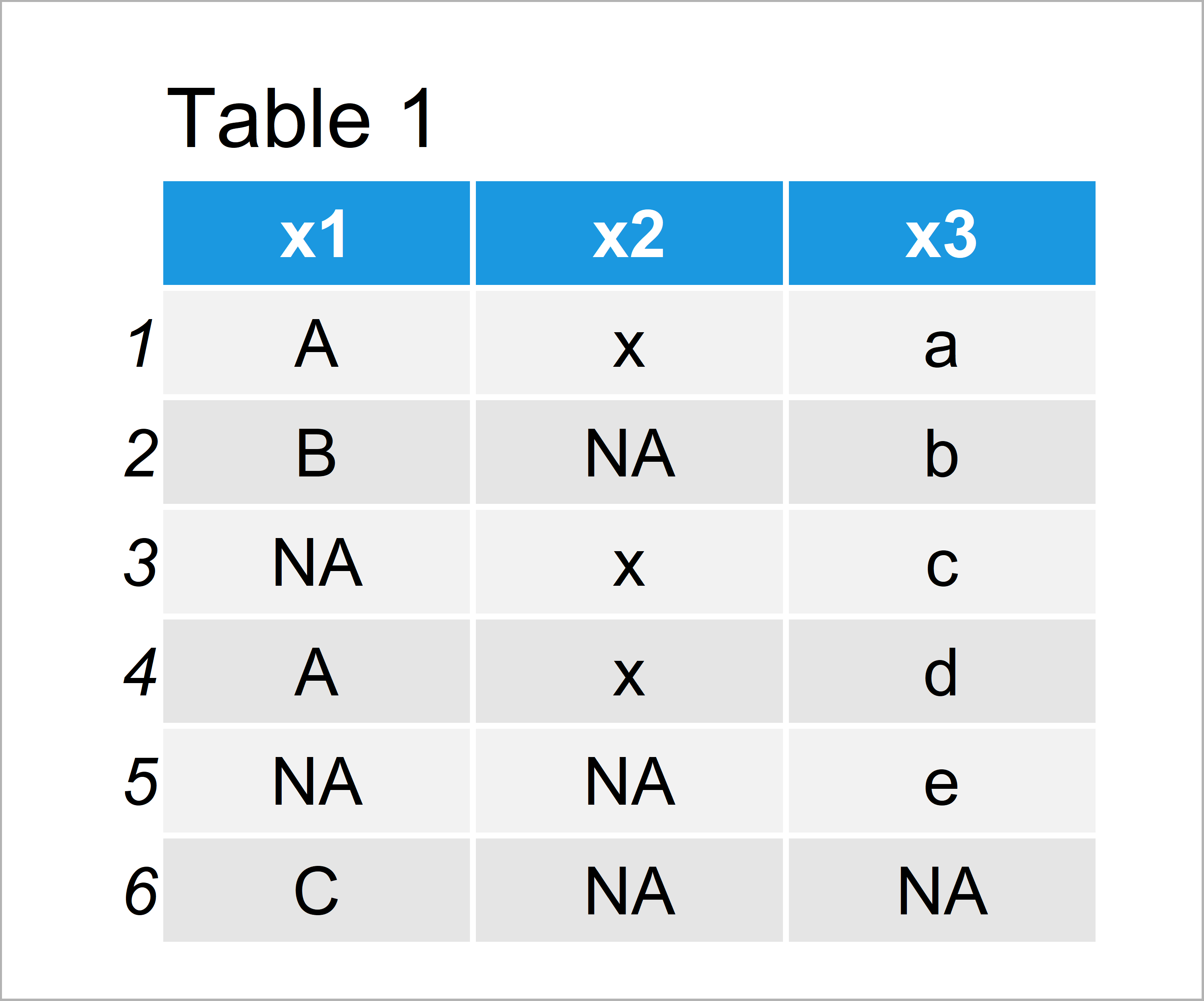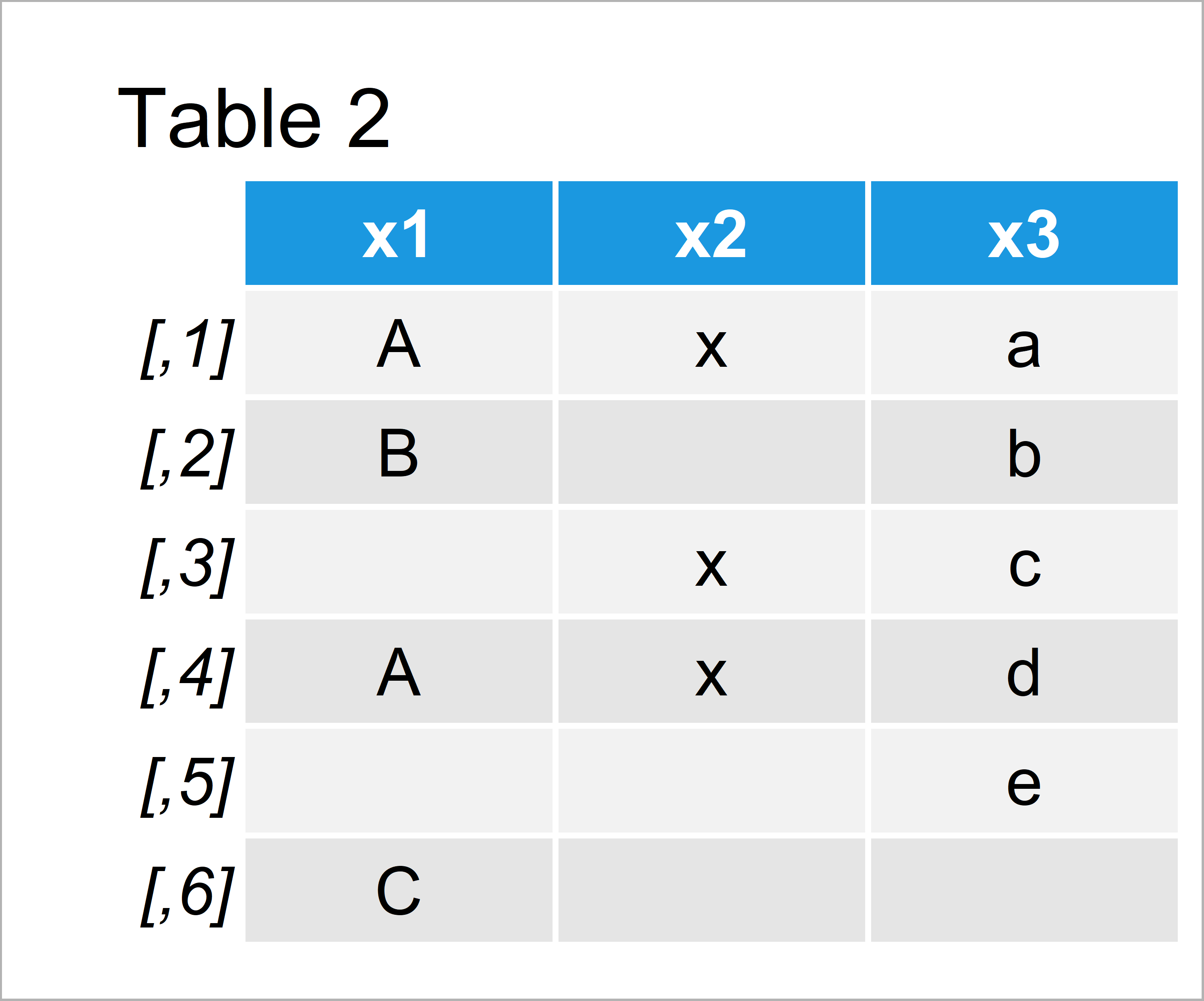# Set NA to Blank in R (2 Examples)

In this article, I’ll explain how to replace NA with blank values in the R programming language.

Let’s take a look at some R codes in action.

## Example 1: Replace NA with Blank in Vector

This example illustrates how to set NA values in a vector to blank.

As a first step, we have to create an example vector in R:

```vec <- c("A", "B", NA, "A", NA, "C") # Create example vector vec # Print example vector #  "A" "B" NA "A" NA "C"```

The previous output of the RStudio console shows that our example vector contains six elements, whereby three of these elements are NA (i.e. Not Available or missing data).

If we want to replace these NA values by blanks, it is important to know the data type of our vector. We can check that by using the class function:

```class(vec) # Check class of vector #  "character"```

Our vector is a character string.

That’s good news, because if the vector would have the factor class we would have to convert it to the character class first. Check out this tutorial for more info.

Now, we can substitute our NA values by blanks using the is.na function:

```vec_blank <- vec # Duplicate vector vec_blank[is.na(vec_blank)] <- "" # Replace NA with blank vec_blank # Print updated vector #  "A" "B" "" "A" "" "C"```

The previous output of the RStudio console shows our updated vector object. As you can see, all missing values were replaced by blank characters (i.e. “”).

## Example 2: Replace NA with Blank in Data Frame Columns

Example 2 illustrates how to substitute the NA values in all variables of a data frame with blank characters.

First, we have to create some example data in R:

```data <- data.frame(x1 = c("A", "B", NA, "A", NA, "C"), # Create example data frame x2 = c("x", NA, "x", "x", NA, NA), x3 = c(letters[1:5], NA)) data # Print example data frame```In Table 1 it is shown that we have created a data frame with six rows and three columns. Each of our columns contains NA values.

In the next step, I’m replicating our data frame. This is important, in case we want to keep an original version of our data:

`data_blank <- data # Duplicate data frame`

Next, we are converting all data frame variables to the character class.

We only have to apply this step in case we have factor variables in our data. However, it can not hurt to apply the following line of code:

`data_blank <- sapply(data_blank, as.character) # Convert all columns to character`

Finally, we can set all NA values in our data frame to blank using the is.na function:

```data_blank[is.na(data_blank)] <- "" # Replace NA with blank data_blank # Print updated data frame```By running the previous R syntax we have created Table 2, i.e. a data frame with blank values instead of missings.

## Video, Further Resources & Summary

Would you like to know more about NA values? Then you may want to watch the following video of my YouTube channel. I show the content of this tutorial in the video:

In addition, you may want to have a look at the related tutorials of my homepage. I have released several tutorials already.

You have learned in this tutorial how to exchange missing data with blank character values in the R programming language. In case you have any further questions, let me know in the comments. Besides that, don’t forget to subscribe to my email newsletter in order to get updates on the newest articles.

Subscribe to my free statistics newsletter